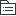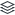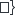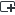# 《极简主义：找到生活的真意》

《极简主义：找到生活的真意》读书笔记分享

97
|
16

## 相关模板推荐

•## 《极简主义》 —— 作品大纲

•健康
• 健康重要性
• 定义健康
• 精神上
• 肉体上
• 如何健康？
• 核心
• 饮食
• 锻炼
• 如何吃
• 给一堆建议多吃的
• 一些待别的饮食方式
• 素食主义
• 严格素食主义
• 鱼素主义
• 石器时代饮食主义
• 给一堆能不吃就不吃的
•吃出健康
• 如何锻炼
• 定义锻炼
• 我们是否在持续改善健康？
• 我们堆积的进步是否满意？
• 锻炼方法
• 净化心灵
• 避免族妒
• 控制压迫感
• 如何做艰难的决定
• 把身边事分一分为二
• 应该做
• 必须做
• 把应该做的事情转换为必须做
•人际关系
• 人际关系最重要
• 定义人际关系
• 被爱
• 分享
• 反思过去的人际关系
• 避免因为他人的观点而烦恼
• 1.询问他们这么做/说是什么意思
• 2.接受事实：不管你怎么问，别人的真图永远无从得知。
• 寻找新关系
• 你要什么
• 避免什么
• 为此你需要做出哪些改变
• 关系八要素
• 信任
• 诚实
• 关怀
• 支持
• 专注
• 可靠
• 理解
• 断掉互联网
• 原因
• 浪费时间
• 没意义
• 好处
• 放弃电视
• 自由了，可以多点事件处理人际关系
•汇流
• 前两个核心很重要
• 后三个也很重要
• 平衡五个核心
• 专注重要事务
• 开始实践
•回顾
•热情
• 热情最重要
• 工作要有热情（而不是只为了挣钱）
• 要有兴趣，有使命
• 热情的枷锁
• 身份
• 在工作中地位
• 地位
• 在社会中
• 确定性
• 避免温水煮青蛙
• 理财
• 预算
• 投资自己
• 避免债务
• 少买没用的
• 奉献
• 努力移除枷锁
• 寻找兴趣点
• 把兴趣变成使命
• 理财
• 预算
• 资源
• 开销
• 没必要的别花钱
• 金钱换自由（没钱就没自由了？....）
• 成长
• 奉献
• 实践
•成长
• 成长最重要
• 要积累
• 寻找杠杆
• 目标明确
• 要立即行动
• 要持续
• 三十堂人生课
•奉献
• 奉献最重要
• 成长是前提
• 奉献会给你增加价值
• 如何奉献
• 参与当地组织，社区
• 做喜欢的事，进行分享
• 满足感
• 正面经历
• 你喜欢的
• 你不喜欢的
• 要给与，才生活
• 捐献不要的东西
• 舍不得，出时间也可以
• 聆听
• 身体力行
• 切莫虚度光阴
•浮动主题